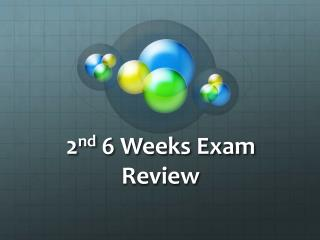DownloadDownload Presentation2 nd 6 Weeks Exam Review

# 2 nd 6 Weeks Exam Review

Download Presentation## 2 nd 6 Weeks Exam Review

- - - - - - - - - - - - - - - - - - - - - - - - - - - E N D - - - - - - - - - - - - - - - - - - - - - - - - - - -
##### Presentation Transcript

1. 2nd 6 Weeks Exam Review

2. Chemical Changes • When a spoon tarnishes is it a chemical change? • Yes • When water melts is it a chemical changes? • No • When fireworks explode is it a chemical change? • Yes • When you mix two substances and there is bubbling and a white substance formed, is it a chemical change? • Yes

3. What is the difference… • Between a PHYSCIAL and CHEMICAL change? Tell me the difference and give an example.

4. Elements vs. Compounds Found in nature Only 1 Made of 2 or more Found on the periodic table O CO H2O Compounds Elements

5. Is it a Compound or Element? • Na • Element • F • Element • HOH • Compound • Ar • Element • NaCl • Compound

6. Metals, Nonmetals, and Metalloids • 1) Metals • 2) Nonmetals • 3) Metalloids • 4) O • 5) Fe • 6) As • 7) Ag • A)Example of Metalloid • B) Conducts electricty and is magnetic • C) Is a semiconductor • D) Example of metal • E) Example of nonmetal • F) Has a low melting and boiling point • G) Is the element silver and a good conductor of electricity

7. How do I find the density? D= M/V • I have a box with a length of 2 meter, a height of 2 meters, and a width of 1 meter. • I know the mass of the box is 288 grams. • What is the density of the box? • 72 l/g • Tell me the steps it took to get to the density.

8. What is the density? • I have a piece of material that is 3 meters wide, 6 meters long, and 4 meters tall. • The object has a mass of 80 kilograms. • What is the density of the piece of material.

9. Lab Equipment • What piece of lab equipment would you use when measuring the force of an object? • What do you use when finding out how much time has elapsed during a part of an experiment? • I want to find the mass of an object. Should I use a beaker or triple beam balance? • When measuring a small amount of liquid what should I use? • When measuring a large amount of liquid what should I use?

10. Safety Equipment • Where is the eye wash station? Is this emergency or preventative safety equipment? • When you are handling a hot beaker, what safety equipment should you wear? • What is an example of a preventative safety equipment? • What is an example of a emergency safety equipment?

11. The Atom (1) Proton (A) Both are in the nucleus (B) Equals the atomic number (2) Neutron (C) Has a positive charge (D) Travels outside the nucleus (E) Has no charge (3) Electron (F) Has a negative charge (G) Equals the mass number • What are the three parts of an atom?

12. Analogies • Positive: Protons :: Negative: _____________ • Electrons • Leaves turning : Chemical change ::__________ : Physical Change color • There is more than 1 right answer…as long as it’s a physical change you are right!

13. What do you think are the most abundant elements in the human body? • Oxygen 65% • Carbon 18% • Hydrogen 10% • Nitrogen 3%

14. States of Matter • Which picture correlates with a SOLID, LIQUID, GAS, and PLASMA? 1. 2. 3. 4. What is the state of Gold? What is the state of Hydrogen? What is the state of Cl?

15. What’s the answer to the question? • A student was given a liquid and solid that produced a gas when mixed together. • Use the table below to find out how much liquid and solid she would need to make 6.3 grams of gas.